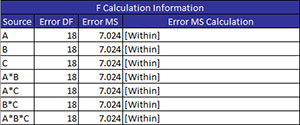# F Value Calculation Information (ANOVA)If the output option “Display F Value Calculation Information” is selected, the program will add information on how the F value was calculated to the ANOVA worksheet.

If your design is a crossed design containing fixed factors only, the F value is easily determined.  In this case F is simply the mean square for the component divided by the mean square error.  However, this is not true when random factors are involved or there are nested designs.  In this case, you have to use the expected mean squares to determine what to divide the mean square for the component by to obtain a F value.

The F calculation information for this example is shown in the table below.There are four columns.

• Source:  source of variation for which the F value is being determine
• Error DF: the degrees of freedom associated with the error term
• Error MS: the mean square error
• Error MS Calculation: the terms included in the mean square error